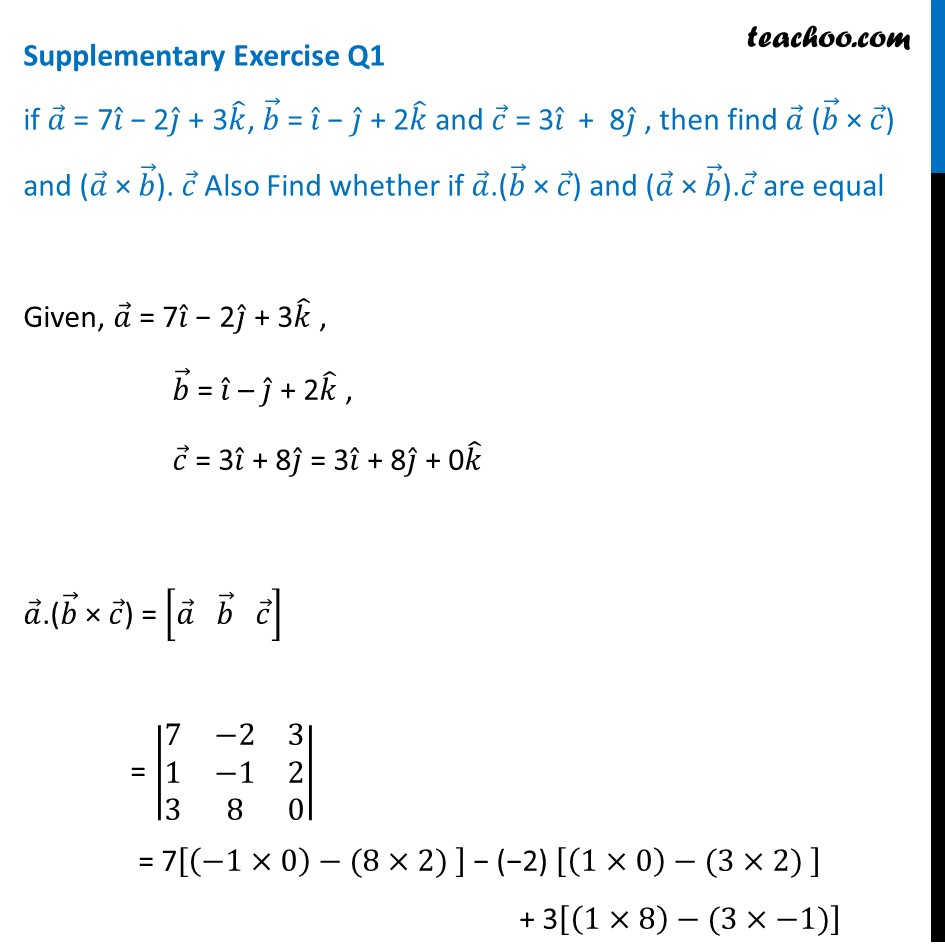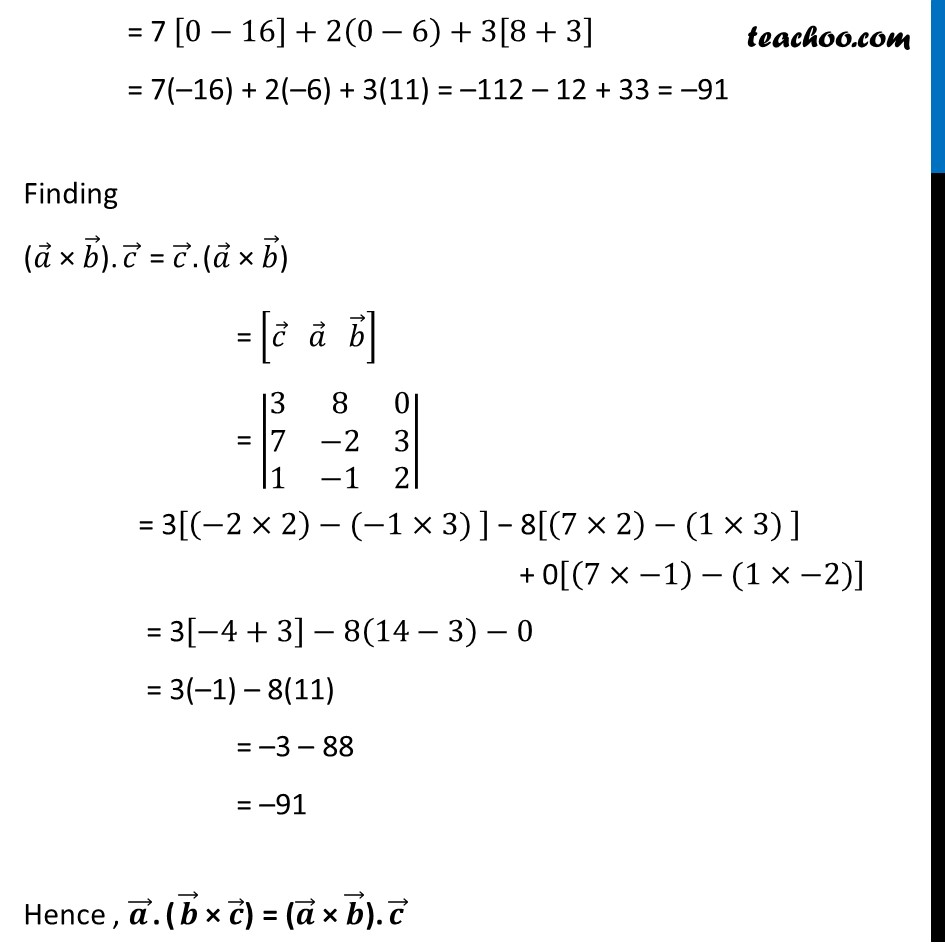Supplementary examples and questions from CBSE

Chapter 10 Class 12 Vector Algebra
Serial order wiseIntroducing your new favourite teacher - Teachoo Black, at only ₹83 per month

### Transcript

Supplementary Exercise Q1 if 𝑎 ⃗ = 7𝑖 ̂ − 2𝑗 ̂ + 3𝑘 ̂, 𝑏 ⃗ = 𝑖 ̂ − 𝑗 ̂ + 2𝑘 ̂ and 𝑐 ⃗ = 3𝑖 ̂ + 8𝑗 ̂ , then find 𝑎 ⃗ (𝑏 ⃗ × 𝑐 ⃗) and (𝑎 ⃗ × 𝑏 ⃗). 𝑐 ⃗ Also Find whether if 𝑎 ⃗.(𝑏 ⃗ × 𝑐 ⃗) and (𝑎 ⃗ × 𝑏 ⃗).𝑐 ⃗ are equal Given, 𝑎 ⃗ = 7𝑖 ̂ − 2𝑗 ̂ + 3𝑘 ̂ , 𝑏 ⃗ = 𝑖 ̂ – 𝑗 ̂ + 2𝑘 ̂ , 𝑐 ⃗ = 3𝑖 ̂ + 8𝑗 ̂ = 3𝑖 ̂ + 8𝑗 ̂ + 0𝑘 ̂ 𝑎 ⃗.(𝑏 ⃗ × 𝑐 ⃗) = [𝑎 ⃗" " 𝑏 ⃗" " 𝑐 ⃗ ] = |■8(7&−2&3@1&−1&2@3&8&0)| = 7[(−1×0)−(8×2) ] − (−2) [(1×0)−(3×2) ] + 3[(1×8)−(3×−1)] = 7 [0−16]+2(0−6)+3[8+3] = 7(–16) + 2(–6) + 3(11) = –112 – 12 + 33 = –91 Finding (𝑎 ⃗ × 𝑏 ⃗).𝑐 ⃗ = 𝑐 ⃗.(𝑎 ⃗ × 𝑏 ⃗) = [𝑐 ⃗" " 𝑎 ⃗" " 𝑏 ⃗ ] = |■8(3&8&0@7&−2&3@1&−1&2)| = 3[(−2×2)−(−1×3) ] − 8[(7×2)−(1×3) ] + 0[(7×−1)−(1×−2)] = 3[−4+3]−8(14−3)−0 = 3(–1) – 8(11) = –3 – 88 = –91 Hence , 𝒂 ⃗.(𝒃 ⃗ × 𝒄 ⃗) = (𝒂 ⃗ × 𝒃 ⃗).𝒄 ⃗